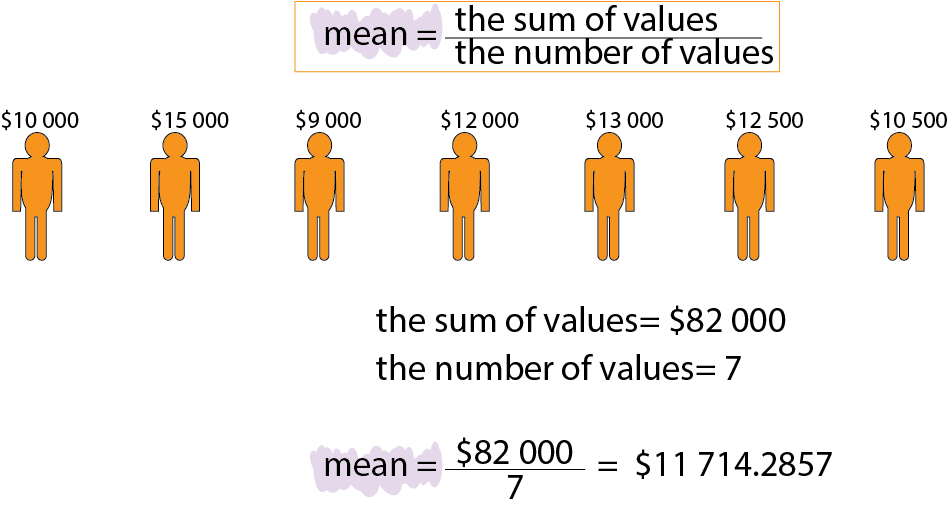Course Content

# Learning Statistics with Python

Learning Statistics with Python

##Mean Value

Now, we will move to the statistical measurements. The first one is called mean value. We can find the mean by adding all numbers in the sequence of data and then dividing by the value quantity.

Look at the picture where we will find the mean value of people's salaries.#You have the list of numbers, find the mean value and replace the gap with it.

`[1, 2, 3]Mean value is `Everything was clear?

Section 1. Chapter 4